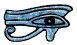# Introduction

One of the most important turning points in the history of modern chemistry was Dalton's Atomic Theory, put forward in 1803. John Dalton (1766 - 1844) was an English chemist who made many other discoveries in chemistry. He was also colour-blind and was the first person to make a scientific study of it; colour-blindness is sometimes called daltonism after him.

Dalton's Atomic Theory says
• Elements consist of indivisible small particles, or atoms.
• All atoms of the same element are identical; different elements have different types of atom.
• Atoms can neither be created nor destroyed.
• Compound elements (compounds) are formed when atoms of different elements join in simple ratios to form compound atoms (molecules).

The words in brackets are the modern terms. At that time only a few elements were known so Dalton originally gave them pictorial symbols, like the symbols for the signs of the zodiac, but as more elements were discovered they were given the present-day symbols of a capital letter or a capital letter followed by a small letter. You can find a list of many of the more common elements and their symbols on The Elements Page of my web site.

Dalton and his contemporaries did not attempt to explain what atoms were, or to estimate their size.

We now know that atoms can be split, and that all atoms of the same element are not identical, and also that a molecule can consist only of atoms of the same element - this is discussed below.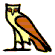# Monatomic and diatomic gases

There are eleven elements which are gases at normal temperature and pressure. Helium, neon, argon, xenon, krypton and radon are the noble gases, and are very unreactive. They exist as single (monatomic) atoms. But hydrogen, nitrogen, oxygen, fluorine and chlorine are very reactive: if they are not joined with atoms of other elements to form compounds they join with atoms of themselves. For this reason they do not usually exist as free atoms but only as diatomic molecules, containing two atoms, for example H2, N2, O2, F2, Cl2. Oxygen also exists as a triatomic molecule O3, and this is given the name ozone.

The early chemists were thrown off course for a time until they discovered that all the reactive gases exist as diatomic molecules.# Mass and Weight

The first person to understand the distinction between the amount of matter in an object and the pull of the Earth on it is usually considered to be the English mathematician Sir Isaac Newton (1642 - 1727). He first began to develop his Theory of Gravity in 1665, when he was just twenty three years old, although he did not publish it until 1697. Today scientists usually refer to the amount of matter in a body as its mass, and the force of gravity on it as its weight. Mass is measured in kilograms, and weight, and all other forces, in newtons. The story is told that Sir Isac Newton first thought of his Theory of Gravity while sitting by an apple tree and watching the apples fall off it. By coincidence a medium size eating apple has a mass of about 100 g so its weight (on the Earth) is about 1 N, but this is not the origin of the newton. At the time of Dalton and his contemporaries however the word weight was used to refer to both the amount of matter in a body and the force of gravity on it (as it is today by most non-scientists, and even many scientists in everyday speech), and in the next Section (but only in the next Section) I shall use weight in this way.# The History of Atomic Weight

Once chemists had realised that chemical reactions took place at an atomic level, and that atoms were neither created nor destroyed, they could start to write symbolic equations. For example, using the modern symbols for the elements,They then worked out, for example, that 4 pennyweight (a unit of mass used by apothocarists in England at this time) of hydrogen always reacted with 32 pennyweight of oxygen to make 36 pennyweight of water; the ratio by weight of hydrogen to oxygen in water was always 1 to 8. So they reasoned that an atom of oxygen weighed 8 times as much as an atom of hydrogen. They already knew that hydrogen was lighter than any other element, so they reasoned that hydrogen atoms were the lightest atoms. So they said hydrogen had an atomic weight of 1. This meant that the atomic weight of oxygen was 8, that is, an atom of oxygen weighed 8 times as much as an atom of hydrogen. Similarly 15 pennyweight of carbon reacted with 40 pennyweight of oxygen to form 55 pennyweight of carbon oxide, so the ratio by weight of carbon to oxygen in carbon oxide was 3 to 8.If the atomic weight of oxygen was 8 the atomic weight of carbon must be 3.

Soon of course it was apparent that something was very wrong, and it was not long before that they realised that water was H2O and carbon (di)oxide was CO2, and that oxygen and hydrogen were diatomic. Using this information they recalculated the atomic weight of oxygen as 16 and that of carbon as 12. Over the next few years they measured the atomic weights of many elements and worked out the chemical formula for many compounds.

Today we use the symbol Ar for the atomic weight of an element. If oxygen has an atomic weight of 16 the correct way of writing this is Ar (O) = 16.

The molecular weight of a molecule is the sum of the atomic weights of the atoms in it. It is given the symbol Mr, so Mr (CO2) = 44 and Mr (H2O) = 18.

It is often regarded as incorrect to refer to a molecule of a substance such as potassium bromide, which has a giant ionic structure, so some people prefer the use of formula weight rather than molecular weight, although the same symbol is used.

The term atomic weight was the term originally used, and it is still widely used today. However, many scientists prefer not to use the word weight in any way other than to mean a force, so use the term relative atomic mass instead. But both atomic weight and relative atomic mass are officially approved by the International Union of Pure and Applied Chemistry, and so are equally correct. But remember that the term atomic weight can only be used for an element not for an isotope.For an isotope we must use relative isotopic mass. (Isotopes are discussed later.)# Atomic weight and the mole

Here is a list of the atomic weights of some common elements, rounded to the values usually used in school chemistry calculations.

• Ar (H) = 1
• Ar (C) = 12
• Ar (O) = 16
• Ar (Na) = 23
• Ar (P) = 31
• Ar (S) = 32
• Ar (K) = 39
• Ar (Ca) = 40
• Ar(Fe) = 56
• Ar(Cu) = 63.5
• Ar (Br) = 80

This means that, to a first approximation, an atom of carbon weighs twelve times as much as an atom of hydrogen, and an atom of bromine weighs eighty times as much. Similarly 20 atoms of carbon weigh 12 times as much as 20 atoms of hydrogen, and 300 atoms of bromine weigh 80 times as much as 300 atoms of hydrogen.  So if we take as many hydrogen atoms as weigh 1 g, the same number of carbon atoms will weigh 12 times as much, that is, 12 g, and the same number of bromine atoms will weigh 80 g. The number of hydrogen atoms that weigh 1 g is the Avogadro constant, symbol L, after the Italian chemist Amedeo Avogadro (1776 - 1856).

Atoms are unimaginably tiny: if we cover a football (soccer) pitch with a layer of sand deep enough to cover the goalposts there are still more atoms in one grain of sand than there are grains of sand on the pitch. The Avogadro constant is about 6 × 1023.

OK, this is a very big number, but nothing to be afraid of; it just means that if you take this number of hydrogen atoms they will weigh 1 g, if you take this number of carbon atoms they will weigh 12 g.

The amount of a substance, any substance, containing L particles is called a mole. A mole of hydrogen atoms contains L atoms and weighs 1 g, a mole of water molecules contains L water molecules and weighs 18 g. A mole of carbon atoms weighs 12 g - this is a small handful of barbeque charcoal. The atomic weight of potassium is 39 so a mole of potassium atoms weighs 39 g. A mole of family-size Christmas puddings would contain L Christmas puddings - and weigh more than the Earth! We must always specify what the particles are, for example a mole of oxygen atoms weighs 16 g but a mole of oxygen molecules weighs 32 g.

If we heat iron filings with sulphur this reaction takes placeOne atom of iron reacts with 1 atom of sulphur to form 1 molecule of iron sulphide, so 100 atoms of iron react with 100 atoms of sulphur to form 100 molecules of iron sulphide, and 1 mole of iron atoms reacts with 1 mole of sulphur atoms to form 1 mole of iron sulphide molecules. A mole of iron atoms weighs 56 g and a mole of sulphur atoms weighs 32 g, so 56 g of iron reacts with 32 g of sulphur to form 88 g of iron sulphide. Similarly if we have a balanced symbolic equation for any chemical reaction we can use the idea of the mole to work out the masses of the chemicals involved.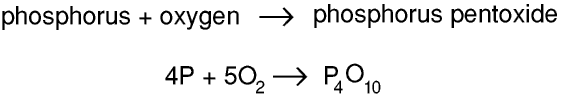Thus 4 atoms of phosphorus react with 5 molecules of oxygen to form 1 molecule of phosphorus pentoxide, so 4 moles of phosphorus atoms react with 5 moles of oxygen molecules to form 1 mole of phosphorus pentoxide molecules, and 124 g (4 × 31 g) of phosphorus reacts with 160 g (5 × 16 × 2 g) of oxygen to form 284 g of phosphorus pentoxide. (It is called phosphorus pentoxide because its formula was originally thought to be P2O5)

There are some more worked examples at the end of this Page.

The above assumes that the unit of atomic mass (the atomic mass unit) is the mass of an atom of hydrogen, which is what it was originally. But today it is taken to be 1/12 of the mass of an atom of carbon-12, so if you want the atomic weight of hydrogen to 4 decimal places it is actually 1.0079, and similarly the atomic weights of all other elements are not whole numbers. This is discussed further below, but this is unlikely to be important to you unless you are studying chemistry beyond GCSE Level or normal school-leaving age. In any exam, at any level, you should always be given the values of all the atomic weights you will need to use to the required accuracy.

The atomic mass unit is also called the unified mass unit. There was a proposal to call it the dalton, after John Dalton (see first paragraph of this Page) but this was never officially taken up although you may come across the term in some older books. The unified mass unit is 1.66033 × 10-27 kg, and is given the symbol u.

Note that the atomic mass unit is given in SI units, that is, kilograms, although the mass of a mole of a substance is always given in grams.# Solutions

We usually measure the concentration of a solution in moles per litre (more correctly, in SI units moles per dm³) or grams per litre of solution. The formula weight of sodium hydroxide is 40, Mr (NaOH) = 40, so a 1 M solution of sodium hydroxide in water contains 40 g of sodium hydroxide per litre. A 1 M solution of sodium hydroxide has a mass concentration of 40 g per litre.

Remember that a 1 M solution contains 40 g per litre of solution not 40 g per litre of water.To make a 1 M solution of sodium hydroxide we take less than a litre of purified water and add 40 g of sodium hydroxide. We stir it well. It will get very hot, so we let it cool to room temperature and then add purified water to bring the volume up to one litre.

The difference between litres and dm³ is discussed in the Density Page of my Web Site, under Density of liquids.

There are some worked examples at the end of this Page.# Gases

If we heat calcium in an open container in air this reaction takes place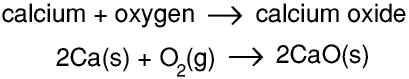Calcium and calcium oxide are solids and oxygen is a gas.

So 2 atoms of calcium react with 1 molecule of oxygen to form 2 molecules of calcium oxide, and 2 moles of calcium atoms react with one mole of oxygen molecules to produce 2 moles of calcium oxide molecules. Hence 80 g of calcium react with 32 g of oxygen to form 112 g of calcium oxide. If we use an ordinary chemical balance to weigh the calcium before the experiment and the calcium oxide after it there seems to be a gain in mass of 32 g because the mass of the oxygen gas has not been measured.

But if we heat calcium carbonate in an open container in air this reaction takes place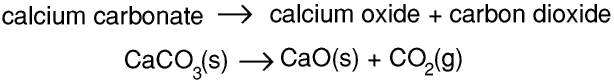1 molecule of calcium carbonate becomes 1 molecule of calcium oxide and 1 molecule of carbon dioxide, so 1 mole of calcium carbonate molecules become one mole of calcium oxide molecules and 1 mole of carbon dioxide molecules.

Calcium carbonate and calcium oxide are solids and carbon dioxide is a gas. 100 g of calcium carbonate produce 56 g of calcium oxide and 44 g of carbon dioxide, that is, this time there seems to be a loss in mass of 44 g.

Until 1766 very few scientists realised that there were gases other than air, or that air was a mixture of gases, or that gases were involved in chemical reactions. It was therefore very difficult for scientists to explain why some substances gained mass when heated in air while others lost mass.

The first gas to be discovered that was obviously quite different from air was prepared in 1766 by the brilliant but eccentric English chemist Henry Cavendish (1731 - 1810). He called this gas inflammable air because it burnt with a blue flame. He found that when it burned it produced water, so demolishing the very old belief that water was an element. As a result the French chemist Antoine Lavoisier (1743 - 1794) renamed it hydrogen, from the Greek for water producer. News of the discovery of hydrogen spread very rapidly through the scientific community, and in 1783 the French Professor Jacques Charles made an ascent in a hydrogen-filled balloon - you can read about this on the History of Ballooning Page.

The next gas to be discovered was oxygen. It was first discovered, in 1772, by the Swedish chemist Carl Scheele (1742 - 1786), and then, independently, in 1774 by the English scientist Joseph Priestley (1733 - 1804). Lavoisier is also given the credit for discovering it, and he named it oxygen, for reasons described below, but his most important contribution was showing that burning involved a chemical reaction, that things needed oxygen to burn, and that the oxygen took part in the chemical reaction, and that it was used up. This represented a breakthrough in the understanding of chemistry the importance of which it is hard for most of us to understand today.

By chance, almost all of Lavoisier’s experiments with oxygen involved non-metals, and the oxides of most non-metals dissolve in water to form acidic solutions. This is why he called the gas oxygen: this comes from the Greek for acid producer. For the next few years it was thought that all acids had to contain oxygen, and this threw them off course for a time when trying to find the formula for hydrochloric acid which does not - its formula is of course HCl.

Almost all substances expand when they are heated, but gases expand a lot more than solids or liquids. Also, all gases are compressible, that is, if you increase the pressure you decrease the volume. So the density of a gas depends upon its temperature and pressure - that is why when you are talking about the density of a gas you must always give the temperature and pressure. Densities of gases are often given at the standard temperature and pressure (s.t.p.) of 0oC and 1013 mb.

Avogadro’s Law states that at the same temperature and pressure the number of particles in a given volume of a perfect gas is the same for all gases. (What is a perfect gas? One for which Avogadro’s Law and the other Gas Laws are true! For most gases these Laws are only an approximation.) At s.t.p. the volume of a mole of hydrogen molecules is about 22.41 dm3. Allowing for the expansion of the gas between s.t.p. and room temperature (usually taken to be 25°C), to a first approximation we often take the density of any gas to be 24 litres per mole (of atoms for the monatomic noble gases, or molecules for all other gases) at room temperature and pressure (r.t.p.). But always remember this is only a rough approximation.

There are some worked examples at the end of this Page.# Relative atomic mass and atomic structure.

All atoms consist of a central nucleus surrounded by one or more electron shells. This is discussed more fully on in the Page on Atomic Structure.

The nucleus of an ordinary hydrogen atom contains just one proton; the nuclei of all other atoms contain both protons and neutrons. The number of protons in the nucleus is called the atomic number and is given the symbol Z. The atomic number determines the type of atom, 1 for hydrogen, 2 for helium, 3 for lithium, etc. The total number of protons and neutrons in the nucleus is called the nucleon number and is given the symbol A. All elements (except fluorine) exist in the form of several isotopes, atoms with the same number of protons (same atomic number) but different numbers of neutrons and so different nucleon numbers. An atom with a particular atomic number and nucleon number is called a nuclide. The commonest isotope of oxygen contain 8 protons and 8 neutrons. We call this nuclide 16O or oxygen-16.

Protons and neutrons have about the same mass, and electrons about 1/1800 of this, so an oxygen-16 nuclide is about 16 times heavier than a hydrogen-1 nuclide, so, to a first approximation, the relative isotopic mass of an oxygen-16 atom is 16. To a first approximation the relative isotopic mass of any nuclide is its nucleon number. The nucleon number A of a nuclide is therefore sometimes called its mass number.

But this is only an approximation because the mass of an electron is not zero, and the mass of a neutron is not exactly the same as that of a proton, and so the relative atomic mass of a nuclide is not exactly its nucleon number (apart from carbon-12 of course, see below). A proton does in fact have a (rest) mass of about 1.007398 u, a neutron about 1.008793 u and an electron about 0.0005486 u, so although the nucleon number of a nuclide must be a whole number the relative isotopic mass will never be, except of course for carbon-12. (Even here you will note that the sum of the masses of six protons, six electrons and six neutrons is not exactly 12 u - but the reason for this is beyond the scope of this Page.)

If we have 100 metal spheres, 75 weighing 35 g and 25 weighing 37 g, the total mass of all the spheres is 75 × 35 + 25 × 37 g or 3550 g, so the mean mass of a sphere is 35.5 g. Similarly in Nature the element chlorine consists of about 75% chlorine-35 and 25% chlorine-37, so the (mean) relative atomic mass of an atom of chlorine is 35.5. All elements (except fluorine) occur in Nature as a mixture of several isotopes, so the mean relative atomic mass of a element is calculated taking into account the proportions of all the isotopes in it. (Today most people leave out the mean.) For an element we should always use the relative atomic mass, or of course atomic weight. Chlorine and copper are notable exceptions, but for most other elements one isotope is much more common that the others so the relative atomic mass of an element is close to the relative isotopic mass (and therefore the nucleon number) of the most common isotope. For example, hydrogen is 99.99% hydrogen-1, carbon is 99% carbon-12 and oxygen is 99.7% oxygen-16, so for many purposes it is sufficiently accurate to take Ar (H) = 1, Ar (C) = 12 and Ar (O) = 16.

The 19th century chemists took the atomic weight of hydrogen to be 1, that is, they took the unit of atomic mass (atomic mass unit or amu) to be the mass of a hydrogen atom. As they became able to measure atomic weight more accurately they discovered that, using an atom of hydrogen as the atomic mass unit, the atomic weight of oxygen was 15.87. As at this time almost all atomic weights were determined directly by doing chemistry experiments, and far more of these experiments involved oxygen than hydrogen, it was decided to take the atomic mass unit to be 1/16 of the mass of an atom of oxygen. So Ar (O) = 16.000. This gave Ar (H) = 1.008

Once isotopes were discovered even this was not good enough, so they changed the definition of the atomic mass unit once again: today it is defined as 1/12 of the mass of a carbon-12 atom. So Ar (12C) = 12.0000. In this system Ar (1H) = 1.007825 and Ar (16O) = 15.99491. For the elements (taking into account the other isotopes, that is, the mean relative atomic mass) the values are Ar (H) = 1.0079, Ar (C) = 12.011, and Ar (O) = 15.999. Not exactly 1, 12 and 16, but still close enough if you only need to work out the mass of oxygen needed to oxidise 100 g of methane in a school science exam....# Worked Examples

You will always be allowed to use your calculator in any chemistry exam so do not try to do anything other than the simplest calculations, such as finding the molacular weight of water, without it. I have written down all the calculations exactly as you would enter them into your calculator but you will probably not need to write the stages down, only the result.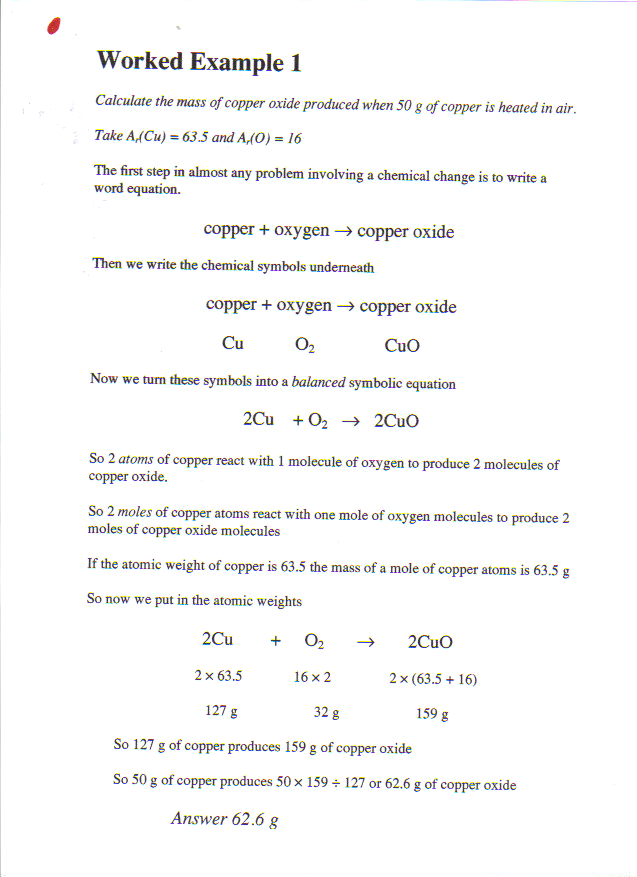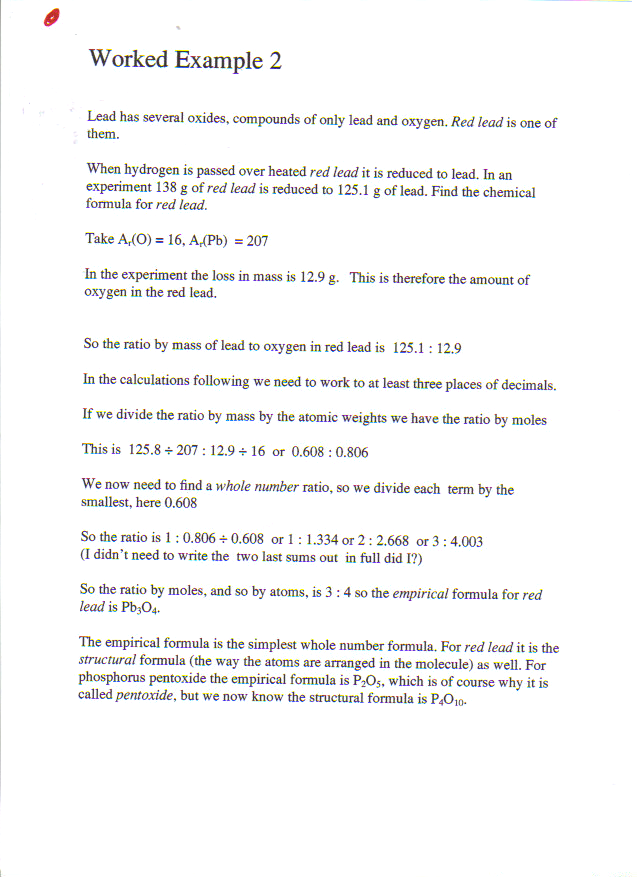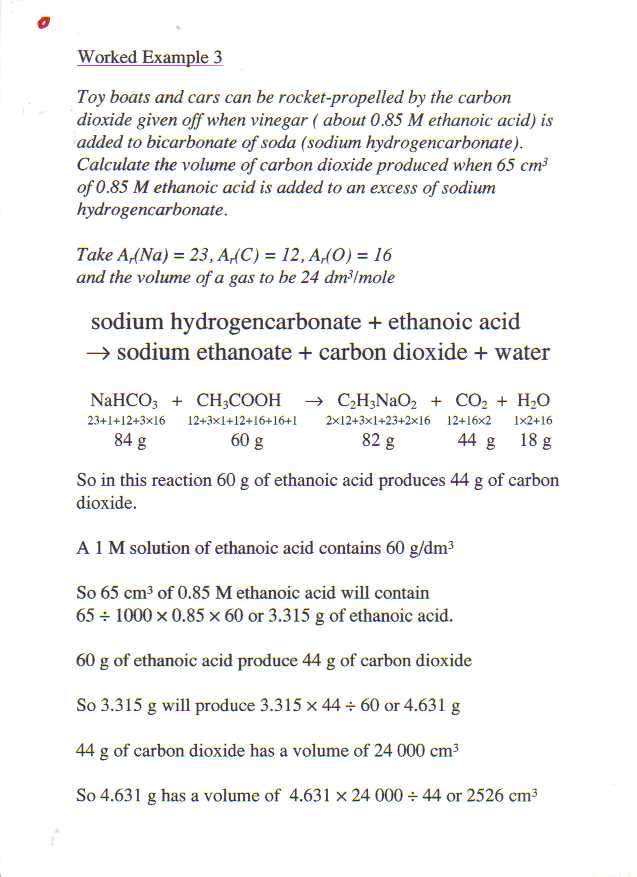© Barry Gray January 2014